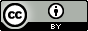“Elementary properties of closures”

From Terence Tao’s Analysis I, p213 of the hardcover second edition, Lemma 9.1.11.

Showing $$X\subset \overline{X}$$. Let $$x\in X$$, and let $$\epsilon >0$$. We have $$\left|{x-x}\right| = 0 \leq \epsilon$$, so we are done.